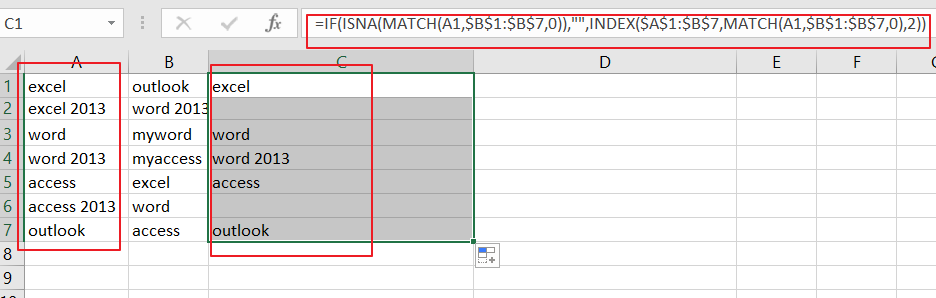# How To Align Duplicate Values within Two Columns in Excel

This post will guide you how to align duplicate values within two columns based on the first column in your worksheet in Excel. How do I use an formula to align two columns duplicate values in Excel.

### Aligning Duplicate Values in Two Columns

Assuming that you have two columns including product names in your worksheet. and you want to find duplicate values in those two columns(Column A and Column B) and align them based on the first column in a new column(Column A and Column B). How to do it. And you can use an formula based on the `IF function`, the `ISNA function`, the `MATCH function`, and the `INDEX function`. Like below:

`=IF(ISNA(MATCH(A1,\$B\$1:\$B\$7,0)),"",INDEX(\$A\$1:\$B\$7,MATCH(A1,\$B\$1:\$B\$7,0),2))`

Type this formula into the first cell in Column C, and press Enter key on your keyboard to apply it. then drag the AutoFill handle down to other cells to apply this formula.Note: Cell A1 is the first cell in the first column, and B1:B7 is the range of cells in the second column.

### Related Functions

• Excel IF function
The Excel IF function perform a logical test to return one value if the condition is TRUE and return another value if the condition is FALSE. The IF function is a build-in function in Microsoft Excel and it is categorized as a Logical Function.The syntax of the IF function is as below:= IF (condition, [true_value], [false_value])….
• Excel INDEX function
The Excel INDEX function returns a value from a table based on the index (row number and column number)The INDEX function is a build-in function in Microsoft Excel and it is categorized as a Lookup and Reference Function.The syntax of the INDEX function is as below:= INDEX (array, row_num,[column_num])…
• Excel MATCH  function
The Excel MATCH function search a value in an array and returns the position of that item.The MATCH function is a build-in function in Microsoft Excel and it is categorized as a Lookup and Reference Function.The syntax of the MATCH function is as below:= MATCH  (lookup_value, lookup_array, [match_type])….
• Excel ISNA function
The Excel ISNA function used to check if a cell contains the #N/A error, if so, returns TRUE; otherwise, the ISNA function returns FALSE.The syntax of the ISNA function is as below:=ISNA(value)….
Related Posts

Break ties with helper COUNTIF and column

Suppose you got a task to adjust the values that contain the ties; what would be your first attempt to break the ties of the given value? If you are wondering about doing this task manually, let me add that ...

Find the Closest Data to the Data Provided in Excel

In our daily work, we may encounter such an issue that to find the closest value to a certain value. In fact, Excel internal functions can help us solve this problem. In today’s article, we will show you how to ...

Abbreviate Names Or Words in Excel

As an MS Excel user, you might have come across a task where you need to abbreviate different names or words, and there are also possibilities that you might have done this task manually by assuming that there isn't any ...

Convert State Names To Abbreviations

Assume that you got a task to convert the full state's name into the abbreviations in MS Excel, and for doing this task, you might choose to do it manually, which is an acceptable way only if you don't have ...

Filter or Remove Columns

Assume that in MS Excel, you have a table consisting of a few columns consisting of few values, and you want to filter to remove the specified columns from the table. You might take it easy and would prefer to ...

Sidebar